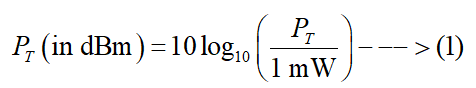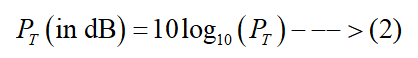# Establish the relation between dB and dBm while measuring the power of an antenna.

Question
1 views

Establish the relation between dB and dBm while measuring the power of an antenna.

check_circle

star
star
star
star
star
1 Rating
Step 1

The dBm is a logarithmic unit of power and it stands for power in decibels when the reference power is one milliwatt. If PT is the power transmitted by an antenna in watts (W), then the power transmitted by the antenna in dBm can be obtained using the following relation:The dB is a logarithmic unit of power and it stands for decibels. If PT is the power transmitted by an antenna in watts (W), then the power transmitted by the antenna in dB can be obtained using the following relation:Step 2

Now, if P is the amount of power to be converted into dBm or dB, then using equation (1) and (2), the following relation can be written:

The meaning of equation (3) is that if the power in a certain experiment is given as 6 dBm, the value of the corresponding power in dB will be 6-30=-24 dB.

...

### Want to see the full answer?

See Solution

#### Want to see this answer and more?

Solutions are written by subject experts who are available 24/7. Questions are typically answered within 1 hour.*

See Solution
*Response times may vary by subject and question.
Tagged in

### Electrical Engineering